# Pi & Fibonacci NumbersRecall that the Fibonacci sequence is defined by F(1) = 1, F(2) = 1, F(3) = 2, F(n) + F(n+1) = F(n+2). The following relation involving the Fibonacci numbers was proven by Ko Hayashi .

(*)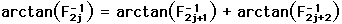The connection to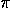is that arctan(1) =/4. Thuscan be expressed in terms of Fibonacci numbers

The first three cases have been demonstrated geometrically using the Geometer's Sketchpad. (These are not really interactive sketches.)• green angle = arctan(1) (look at the 1x1 square)
• red angle = arctan(1/2) (look at the tilted 1x2 rectangle)
• blue angle = arctan(1/3) (look at the 1x3 rectangle)

One can easily see that green angle = red angle + blue angle.
Thus we have ¼/4 = arctan(1) = arctan (1/2) + arctan(1/3).

• arctan(1/3) = arctan(1/5) + arctan(1/8)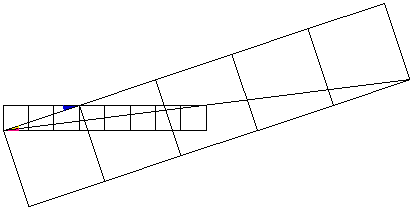As it is difficult to see the angles involved here, the following picture zooms in on the important angles.• blue angle = arctan(1/3) as before (look at a 1x3 rectangle)
• yellow angle = arctan(1/5) (look at the tilted 1x5 rectangle)
• pink angle = arctan(1/8) (look at the 1x8 rectangle)

Again, it is easy see that blue angle = yellow angle + pink angle.
Thus we have arctan(1/3) = arctan (1/5) + arctan(1/8).
Combining this with the previous result yields ¼/4 = arctan(1) = arctan (1/2) + arctan (1/5) + arctan(1/8).

• arctan(1/8) = arctan(1/13) + arctan(1/21)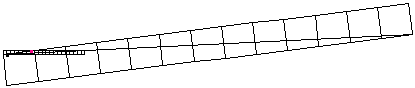It is extremely difficult to see the angles involved here, so again the following picture zooms in on the important angles.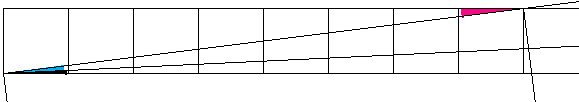• pink angle = arctan(1/8) as before (look at a 1x8 rectangle)
• cyan angle = arctan(1/13) (look at the tilted 1x13 rectangle)
• black angle = arctan(1/8) (look at the difficult to see 1x21 rectangle)

Again, it is easy see that pink angle = cyan angle + black angle.
Thus we have arctan(1/8) = arctan (1/13) + arctan(1/21).
Combining this with previous results yields ¼/4 = arctan(1) = arctan (1/2) + arctan (1/5) + arctan (1/13) + arct an(1/21).

One can representas the sum of an arbitrary number of terms involving Fibonacci numbers by continuing in this manner. The repeated application of equation (*) yields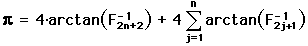, for any natural number n.

Each sum starting with n =1, is an exact representation of. One can look at four times the summation to be a partial sum with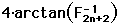as the error term for. The sequence of these partial sums converges toalso. I.e.,.

Using the formula for the tangent of the sum of two angles, these relationships can be easily verified.http://www.geom.umn.edu/~huberty/math5337/groupe/fibonacci.html